Why VIKTOR

Resources

Company

# Extract PLAXIS results automatically using Pythonby Philip Chin Fung Tsang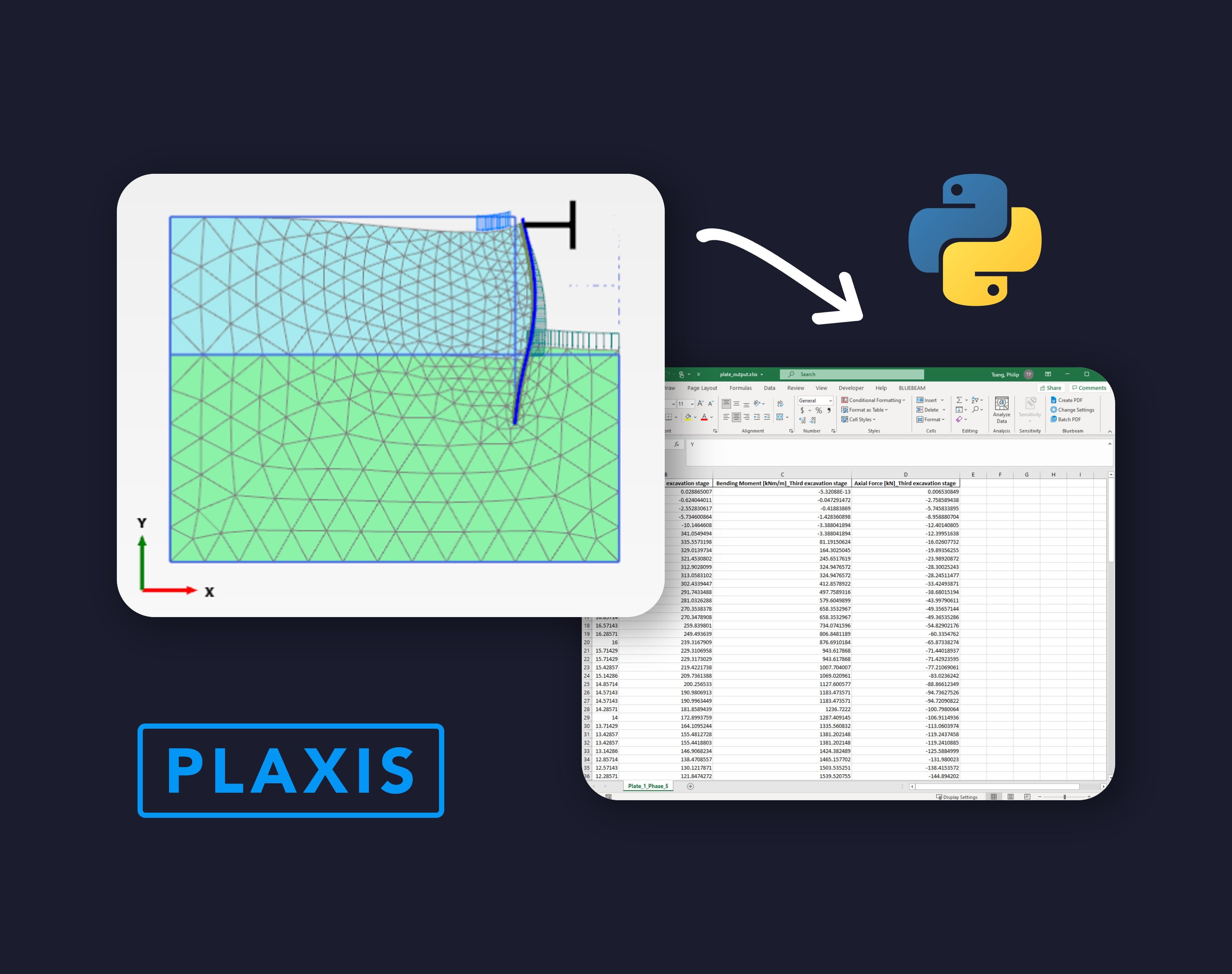If you are a frequent PLAXIS user like me, you must have asked this question: why on earth I need to keep repeating this copy-and-paste action to extract results?##### Build successful applications

Learn how you (developer, engineer, end-user, domain expert, project manager, etc.) can contribute to the creation of apps that provide real value to your work.

PLAXIS, without a doubt, is a powerful FE software. As Geotechnical engineers, we want to focus on the analysis and spend less time on post-processing. Python provides the most extensive possibilities for automating output extraction and visualisation, thanks to the modules developed by the open-source communities. Some common modules include:

• Pandas
• Xlsxwriter
• Matplotlib
• Seaborn

## Goal

The ultimate goal of this tutorial is to show you how to use Python script to extract outputs from a structural element in PLAXIS 2D. This can be done with the following four steps.

1. Use IDE to open PLAXIS Output
2. Install external module to PLAXIS environment
3. Extract Plate results from PLAXIS

This tutorial requires the readers have VS Code and PLAXIS environment installed. Follow the instructions from the article below if you're new to this page. Let's get started!

Start Using Python to Automate PLAXIS

## PLAXIS Example Model

I used a PLAXIS 2D model published by the official Bentley website as shown below.

In the link, download the command log file from V22.01. To the best of the author's knowledge, the Python commands have changed significantly from V21 to V22. Hence, make sure you got PLAXIS V22 or above (V22.01 and V22.02) installed to ensure the Python script in this tutorial works properly.

PLAXIS 2D Tutorial 03: Submerged construction of an excavation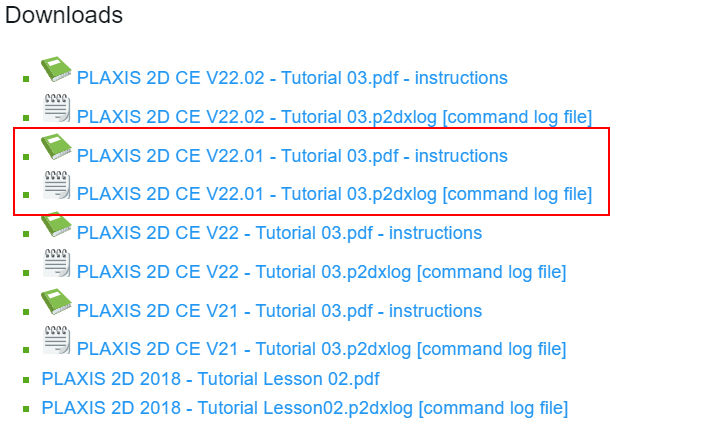Once you have run the command using "Run commands...", you should be able to see the following geometry.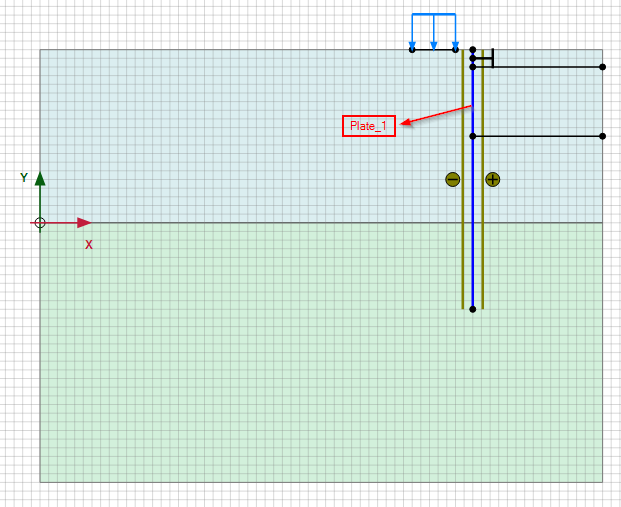This example models the construction of an excavation which consists of a diaphragm wall (Plate element). Our goal is to extract the results of the plate element.

Calculate the model and we are ready to extract the results using Python.

## Step 1: Use IDE to Open PLAXIS Output

Unlike first tutorial, this time we will open a PLAXIS file we just downloaded and hence the code for starting the server needs to be slightly modified. You will need to save the calculated model in a preferred working directory.

My location of the PLAXIS file:

I will first create an empty python script called "open_output.py".

After that, we write the code below to the python file. The code is very similar to the one from the first tutorial but this time we will be opening an existing PLAXIS file. In order to do this, we made the following three changes:

1. Since this time we need to open a PLAXIS file, the working directory of the file needs to be specified which is stored as "FILE_PATH".
2. We then open the file using PLAXIS command "s_i.open(FILE_PATH)".
3. To open PLAXIS output, we write "g_i.view(g_i.Phases)" which allows us to view the results of Phase_1's calculation.
``````1from plxscrpting.easy import *
2import subprocess, time
3import os
4
5
6###############################################
7PLAXIS_PATH = r'C:\Program Files\Bentley\Geotechnical\PLAXIS 2D CONNECT Edition V22\\Plaxis2DXInput.exe'  # Specify PLAXIS path on server.
8FILE_PATH = r'C:\Users\phtsang\Desktop\Current_Projects\LinkedIn\Blog_2\\Excavation'  # Specify PLAXIS file location and name
9PORT_i = 10000  # Define a port number.
10PORT_o = 10001
12
13
15time.sleep(5)  # Wait for PLAXIS to boot before sending commands to the scripting service.
16
17
18# Start the scripting server.
19# global g_i, s_i
22
23
24s_i.open(FILE_PATH)
25
26
27g_i.gotostages()
28g_i.view(g_i.Phases)``````

To test that the code above is working properly, we will run "open_output.py" in the terminal as shown below. Type "python open_output.py" and click Enter.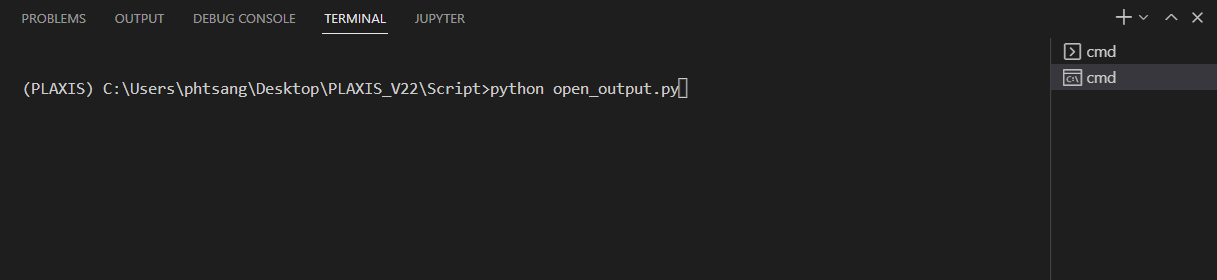Both PLAXIS 2D input and output apps should be opened automatically. From the output app, you should see "SERVER ACTIVE on port 10001".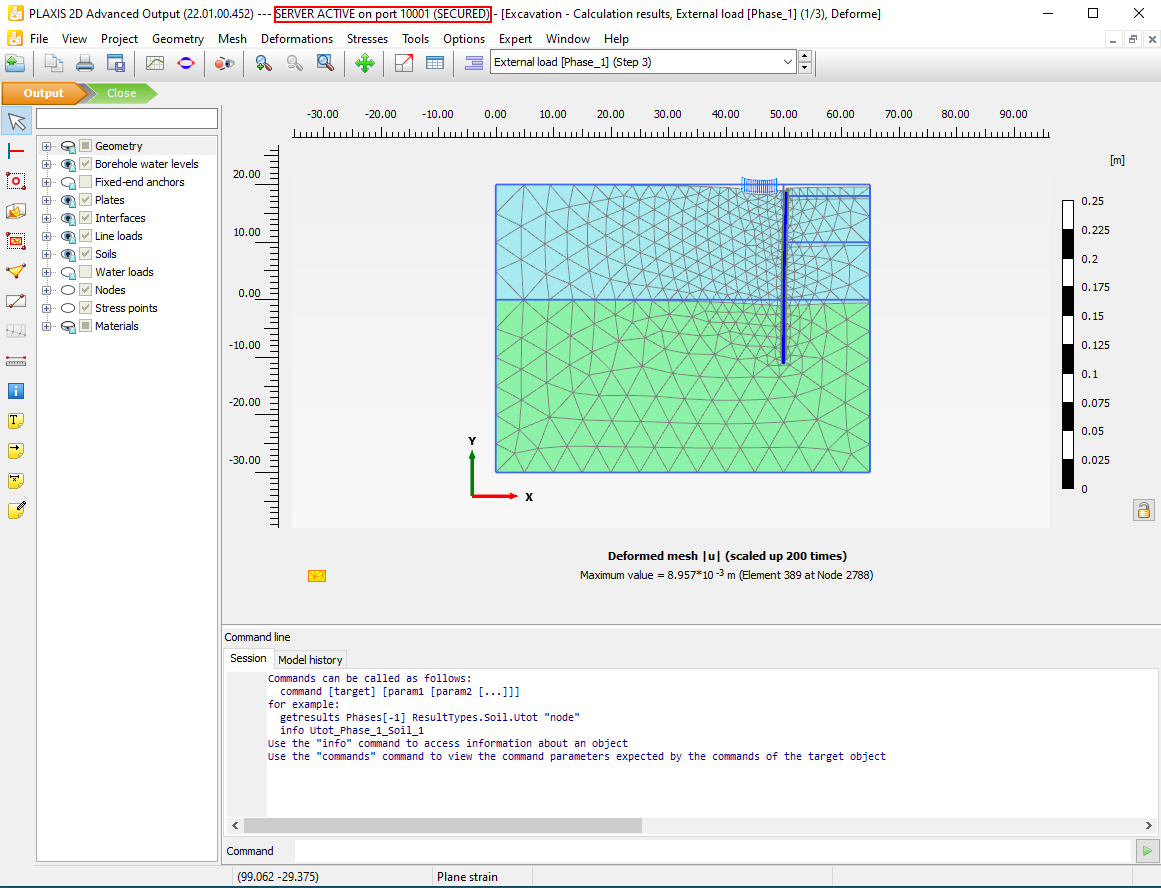We now have the PLAXIS API connected, we are ready to move to next step.

## Step 2: Install External Module to PLAXIS Environment

When using the PLAXIS scripting environment it is common to use Python modules allowing for extending the functionality of a Python script. Since our goal is to extract results from PLAXIS and export to excel spreadsheet, we need two external modules:

• Pandas
• Xlsxwriter

These modules can be installed through PLAXIS command prompt window as shown in the steps below. Before proceeding, make sure you have gone through Step 1 with PLAXIS Input opened.

Go to the menu option Expert > Python > Command prompt. This will open the Windows Command prompt with the PLAXIS set as the current environment.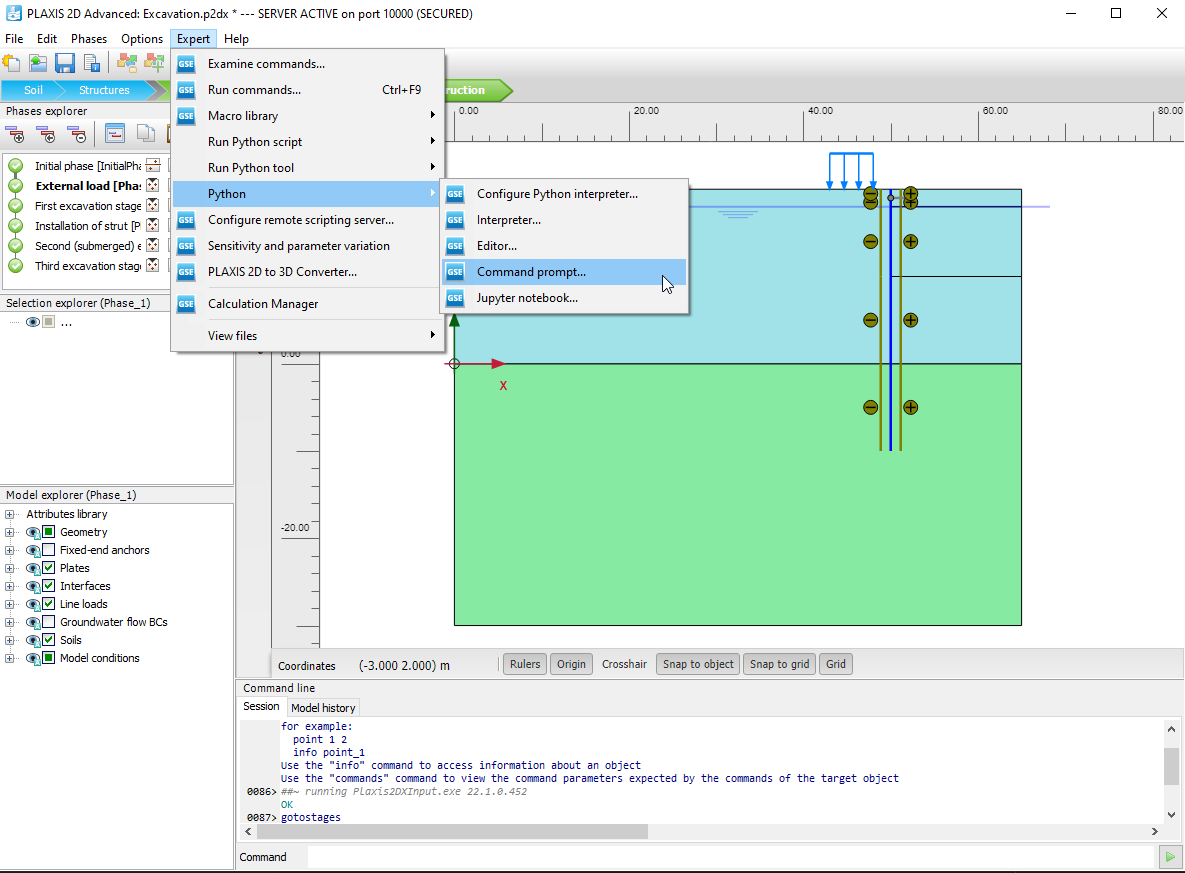Change the directory to the PLAXIS Python Distribution folder. This can be done with the following command:

``1pushd C:\ProgramData\Bentley\Geotechnical\PLAXIS Python Distribution V2\python\Lib\site-packages``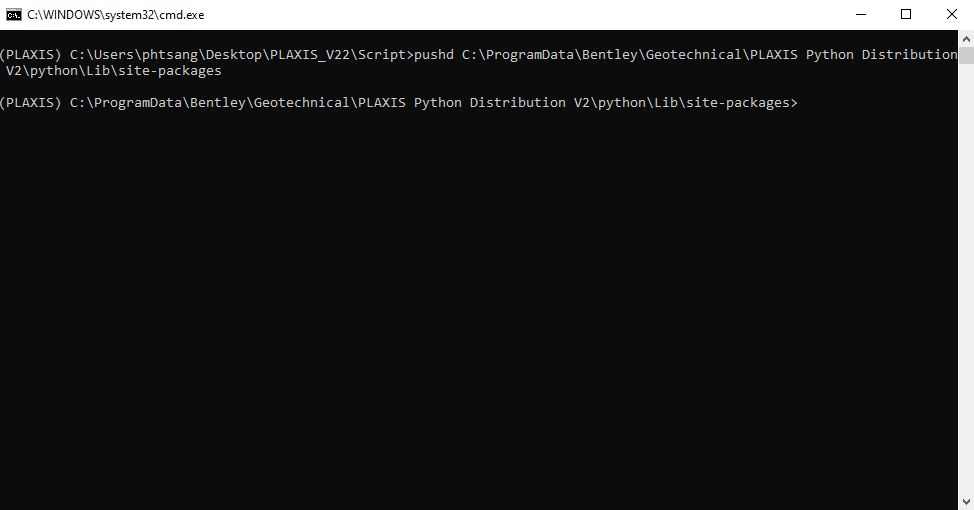In the Command prompt you can now install any new Python modules using the command shown in the example below.

For instance, if you want to install the pandas module:

``1python -m pip install pandas``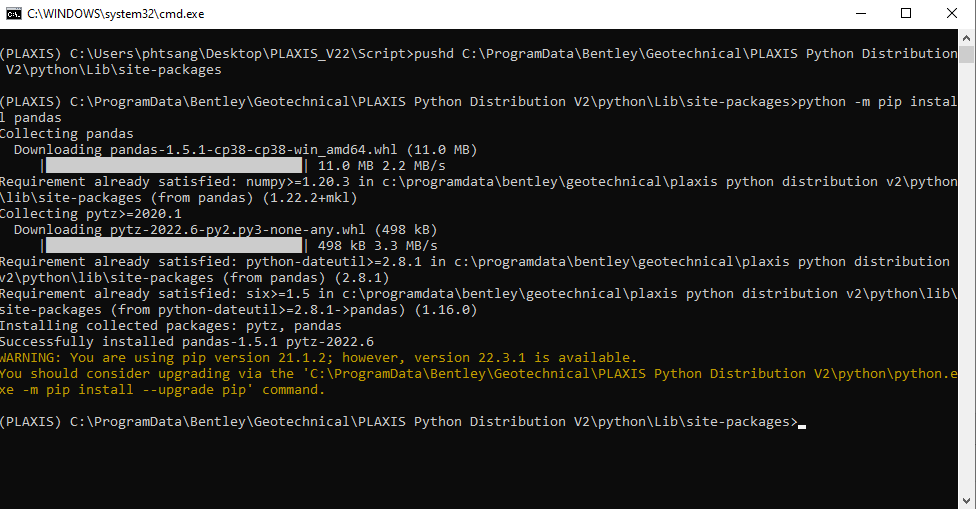Also, install the xlsxwriter module:

``1python -m pip install xlsxwriter``

Now that we have installed all the required modules. We can then extract results from PLAXIS using Python script.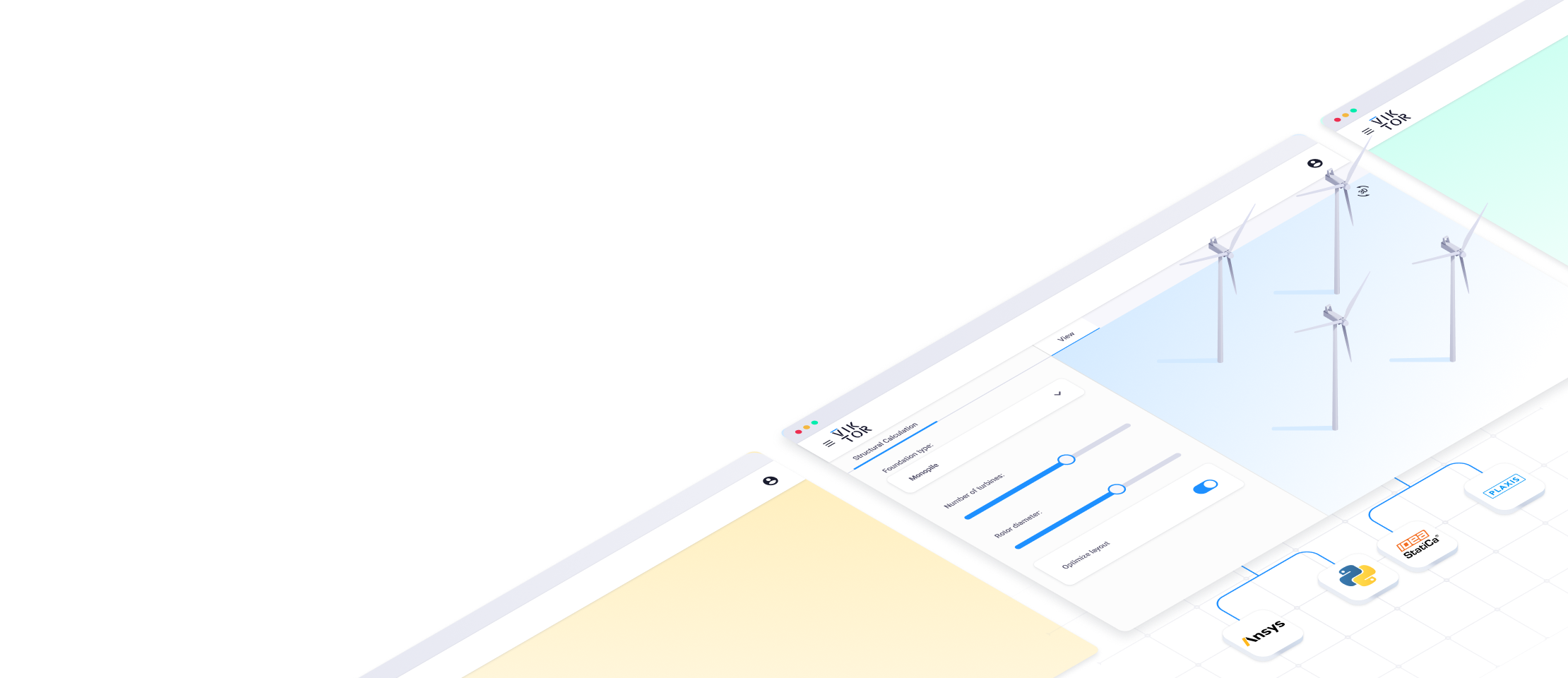## Step 3: Extract Plate Results from PLAXIS

Our main goal Step 3 is to extract four result types for the plate element (Diaphragm wall) in the last phase (Third excavation stage [Phase_5]). The result types we will extract are:

• Y Coordinates
• Shear
• Bending Moment
• Axial Force

In Step 4, we will then export the results to excel spreadsheet with the columns shown below.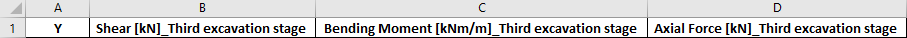First, we create an empty python file and call it "output_plate.py".

### Import Modules and Strat Server

We need to make sure the API is connected, as well as importing the modules we installed earlier.

``````1from plxscripting.easy import *
2import subprocess, time
3import math
4import pandas as pd
5import os
6import xlsxwriter
7
8
9###############################################
10PORT_i = 10000  # Define a port number.
11PORT_o = 10001
13
14
15
16# Start the scripting server.

### Define File Name

Since we need to export the results to excel, we need the specify the exported file location and its name.

File location: make sure there is double slash (\) after your working directory

File name: can be any name you want

``````1EXCEL_PATH=r'C:\Users\phtsang\Desktop\PLAXIS_V22\Script\\'
2EXCEL_NAME='plate_output.xlsx'
3
4FILENAME=EXCEL_PATH+EXCEL_NAME
``````

### Input Definition

After that, we will input the structural element and the corresponding phase we would like to extract. In this case: 'Plate_1' and 'Third excavation stage [Phase_5]'.

The code underneath is to find the existing plate elements and phases in the model and store them as a list.

``````1plate_input=['Plate_1'] # Input by user
2phase_input=['Third excavation stage [Phase_5]'] # Input by user
3
4###############################################
5#Exisiting model element:
6
7plate=[plt for plt in g_o.Plates[:]] # Loop through the plate object in existing model
8phase=[p for p in g_o.Phases[:]] # Loop through all available phases``````

Two important concepts here:

For loop: the action of finding existing elements in PLAXIS requires for loop. This is a very common command in programming. For example in "plate", we have:

``1for plt in g_o.Plates[:]``

This line means we are looping through all the plate elements in "g_o.Plates[:]" (which is how PLAXIS stores objects) and naming it as "plt".

List: As mentioned in the first tutorial, it is common to store values in a list which is represented by a square bracket [ ]. The syntax has slightly changed compared to the first tutorial as it has been simplified.

This line:

``1plate=[plt for plt in g_o.Plates[:]]``

Can be extended as:

``````1plate=[]
2
3for plt in g_o.Plates[:]
4
5   plate.append(plt)
``````

Both code will give the same result but the first one is much neater and is commonly used in Python.

So far, your script should look like: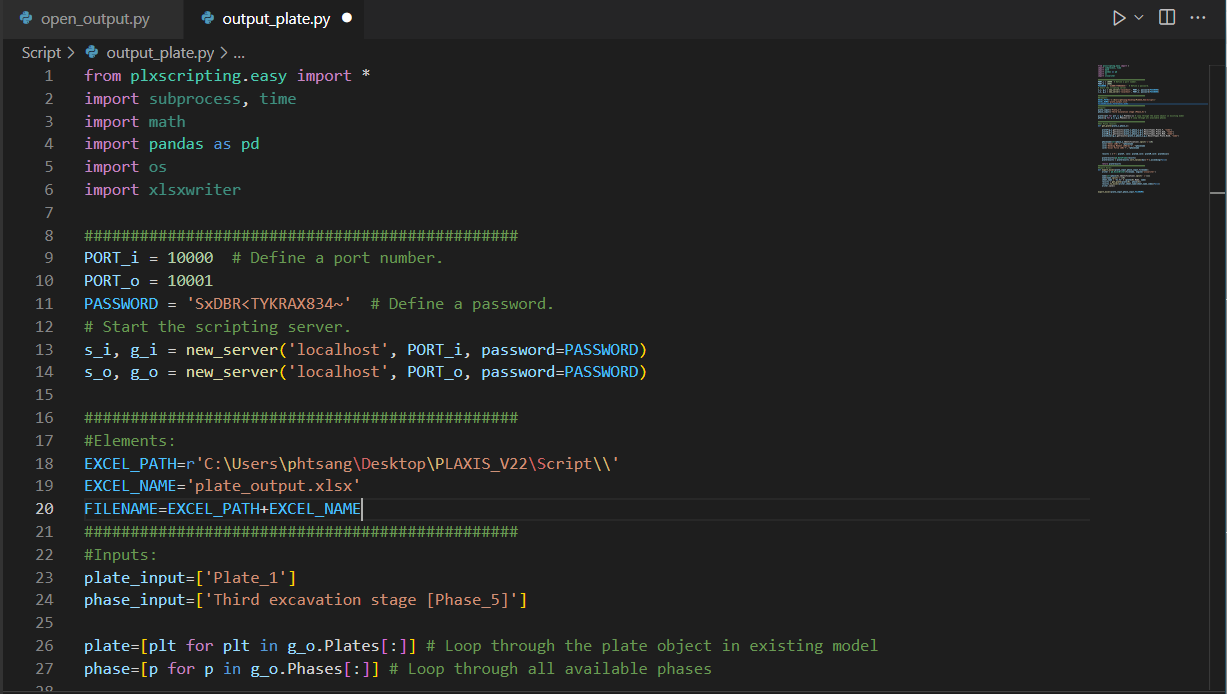### Result Extraction

After the pre-processing as mentioned above, we can start extracting plate results. To do that, we will define a function to extract results, call is "get_plate()". Few key points here:

• "g_o.getresults()" is the PLAXIS command we use to extract results. It takes in plate element, the corresponding phase and the result type you look for. PLAXIS has its own syntaxes for different result types. In our case:

Y coordinate = ResultTypes.Plate.Y

Shear = ResultTypes.Plate.Q2D

Bending Moment = ResultTypes.Plate.M2D

Axial Force = ResultTypes.Plate.Nx2D

• The next few lines are to create column names as specified in the target spreadsheet. Results are then stored as "results". Here I introduce a new data type called Dictionary in Python which is represented by curly bracket { }. Dictionary is particularly useful when you want to create a table, it contains a key-value pair. For example:

'Y' is the key of dictionary

plateY is the value of dictionary. An array of values can be stored to the specified key.

• Once the dictionary is created, the next line convert dictionary to dataframe. Dataframe is one of most common data structure we use in Python. You can interpret it as a table in excel. This is done by running command: "pd.DataFrame(results)"

• I also sort the results using Y coordinate in a descending order

Make sure the indentation is correct as shown in the screenshot below.

``````1def get_plate(plate_o,phase_o):
2
3    plateY=g_o.getresults(plate_o,phase_o,g_o.ResultTypes.Plate.Y, "node")
4    plateQ=g_o.getresults(plate_o,phase_o,g_o.ResultTypes.Plate.Q2D, "node")
5    plateM=g_o.getresults(plate_o,phase_o,g_o.ResultTypes.Plate.M2D, "node")
6    plateAxial=g_o.getresults(plate_o,phase_o,g_o.ResultTypes.Plate.Nx2D, "node")
7
8    phasename=str(phase_o.Identification).split('[')
9    col1='Shear [kN]'+'_'+phasename
10    col2='Bending Moment [kNm/m]'+'_'+phasename
11    col3='Axial Force [kN]'+'_'+phasename
12
13    results = {'Y': plateY, col1: plateQ,col2: plateM,col3: plateAxial}
14
15
16    plateresults=pd.DataFrame(results)
17    plateresults = plateresults.sort_values(by=['Y'],ascending=False)
18
19
20    return plateresults``````

## Step 4: Export As Excel Spreadsheet

Lastly, we will export the dataframe we created to excel.

We create a function, and call it "export_excel()".

• writer is to use the xlsxwriter module we installed to export excel
• name & sheet_name is where I create a worksheet name with format "Plate_1_Phase 5".
• Then, I use the function "get_plate()" we created in earlier to generate the dataframe and store as "results". It should be a dataframe which can then be export to excel using pandas command "results.to_excel()".
``````1def export_excel(plate_input,phase_input,filename):
2    writer = pd.ExcelWriter(filename, engine='xlsxwriter')
3
4
5    name=str(phase.Identification).split(' [')
6    name=name.split(']')
7    sheet_name = "%s_%s" % (plate.Name, name)
8    results = get_plate(plate, phase)
9    results.to_excel(writer,sheet_name=sheet_name,index=False)
10    writer.save()
11
12
13export_excel(plate_input,phase_input,FILENAME)
``````

Run the script with the following.

``1(PLAXIS) C:\Users\phtsang\Desktop\PLAXIS_V22\Script>python output_plate.py``

You will see the results we look for have been automatically extracted as shown in the command window.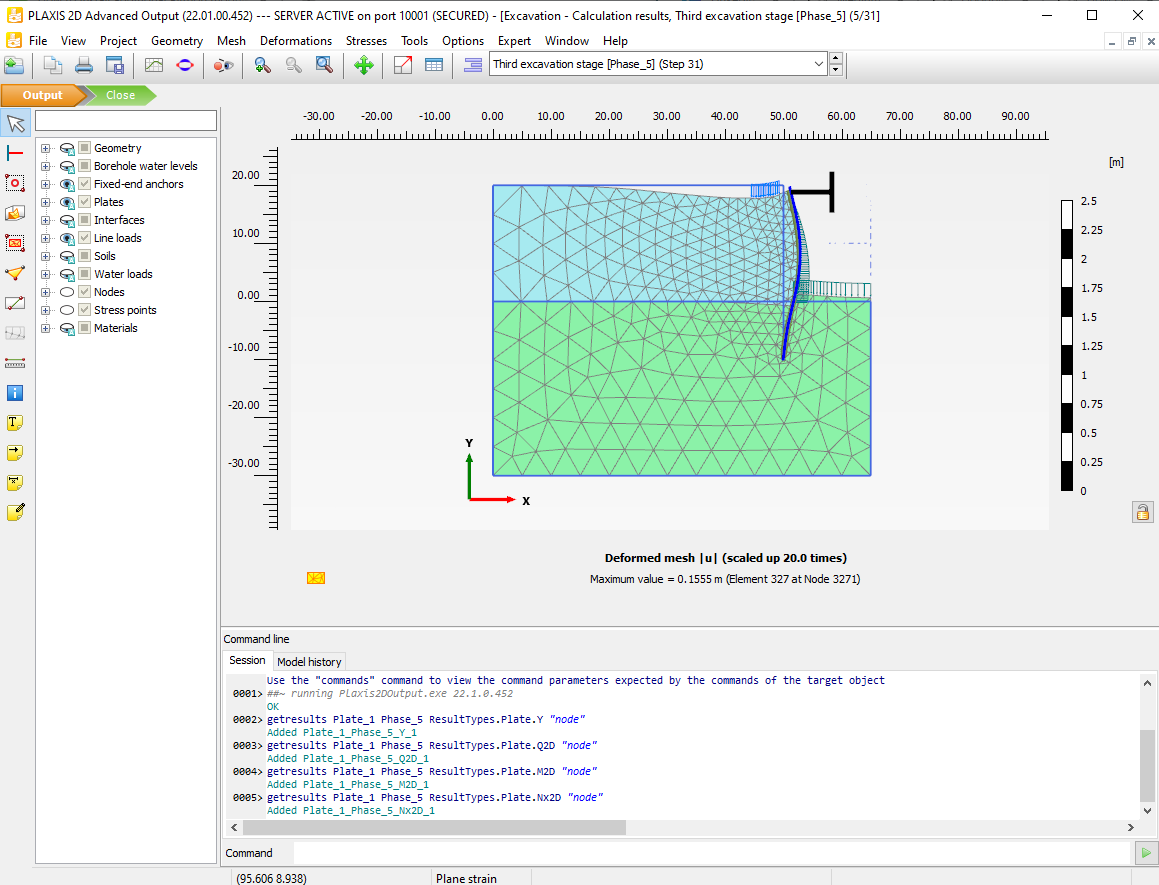Now if you open the excel spreadsheet in the location you specified earlier, you can see we have extracted the Y coordinate, Shear, Bending moment and Axial force as we want.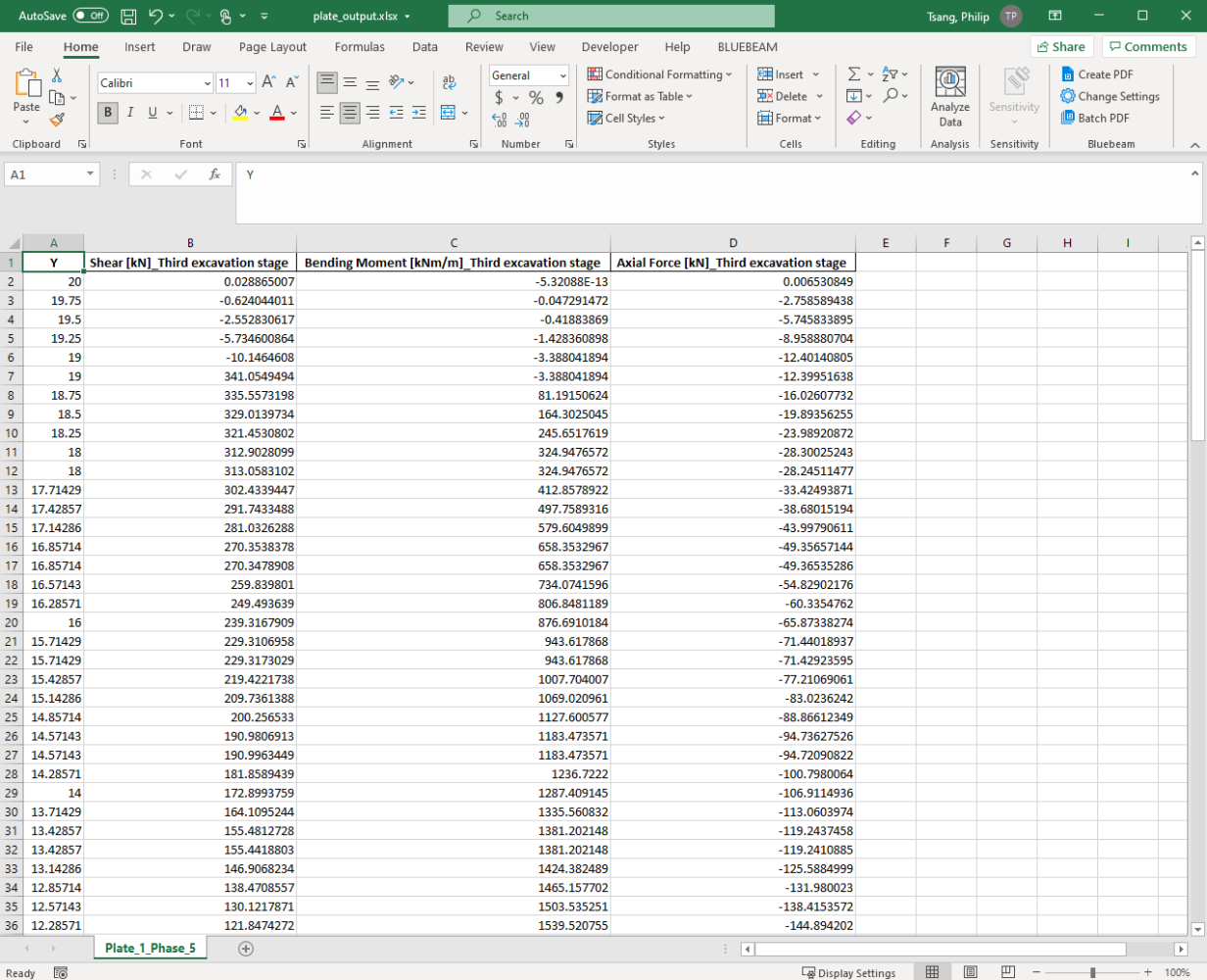Well done! You have just extracted results from PLAXIS using Python script.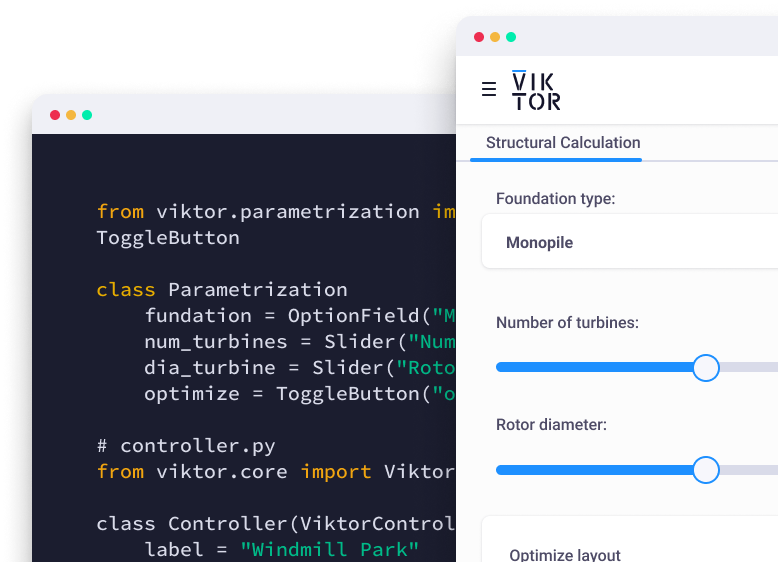Start now

## What's next

That's all for the second tutorial on extracting PLAXIS output using Python. By now, you should be able to extract output for a single structural element from PLAXIS. In future tutorials, I will show how to extract results for multiple structural elements and different structure types such as interfaces and embedded beams.

Here you can find all tutorials to keep improving your automation skills:

Tutorial 1: Start Using Python to Automate PLAX

Tutorial 2: Extract PLAXIS results automatically using Python

Tutorial 3: PLAXIS result visualization using Python

Tutorial 4: Create a PLAXIS model based on a Excel sheet using Python

If you're interested in hearing more about Python, PLAXIS and workflow automation, feel free to follow my LinkedIn page. You can also support me by following me on Medium where I will provide source code and more general programming techniques in the future.Share

## Related Blog Posts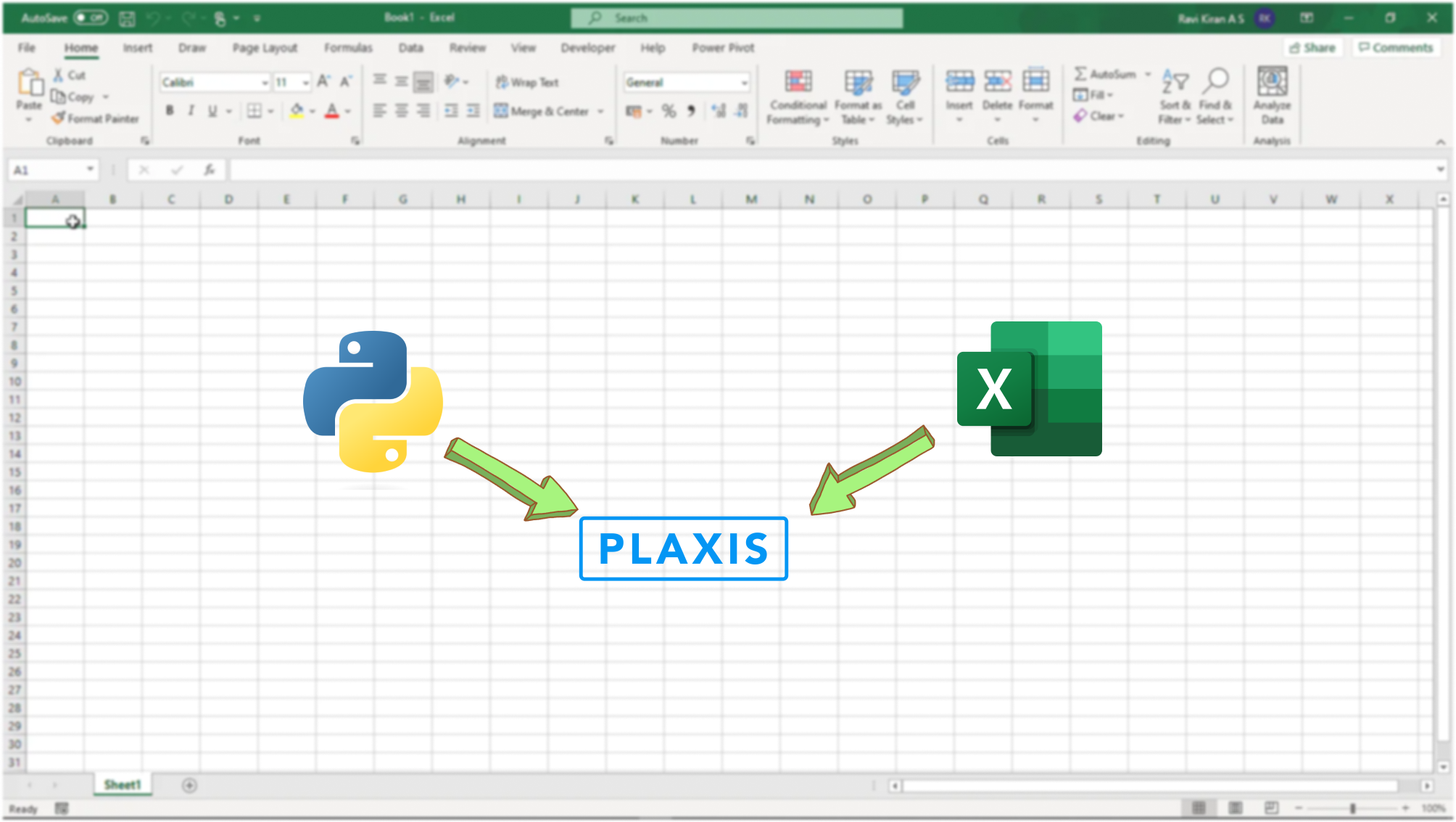### Create a PLAXIS model based on a Excel sheet using Python### Can the AI chatbot ChatGPT do Geotechnical Engineering?